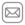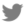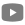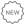||MolLogP (octanol/water partition coefficient)

• Tranining set: 13228 compounds from the PHYSPROP database.
• Descriptors: ECFP4 counted,
• Machine Learning Method: PLS-regression,
• Performance: R2=0.98,Q2=0.95

MolLogS (water solubility Log(Mol/L))

• Tranining set: 1311 compounds from the PHYSPROP database.
• Descriptors: ECFP4 binary+MolLogP,
• Machine Learning Method: Random Forest Regression
• Performance: R2=0.98,Q2=0.86

MolPSA (Molecular Polar Surface Area (PSA) and Volume)

PSA is defined as sum of surfaces of oxygens, nitrogens and attached hydrogens.

• Tranining set: 6K compounds from the WDI database.
• Descriptors: Custom Linear fingerprints
• Machine Learning Method: PLS-Regression
• Performance: R2=1.0,Q2=0.99

Drug-likeness score

Predicts an overall drug-likeness score using and Molsoft's chemical fingerprints. The training set for this mode consisted of:

• 5K of marketed drugs from WDI (positives)
• 10K of carefully selected non-drug compounds. (negatives)

Definitions:

• R2 - squared correlation coefficient of predictions vs. training values
• Q2 - cross-validated squared correlation coefficient of predictions vs. training values